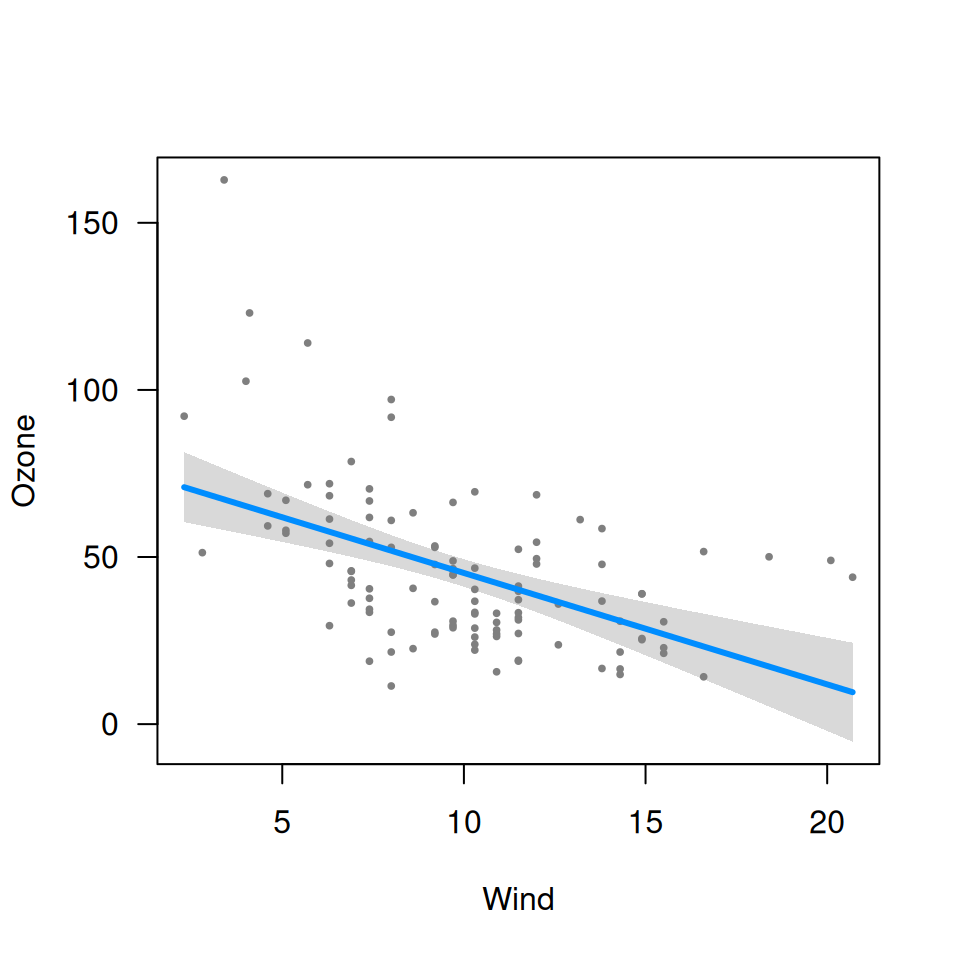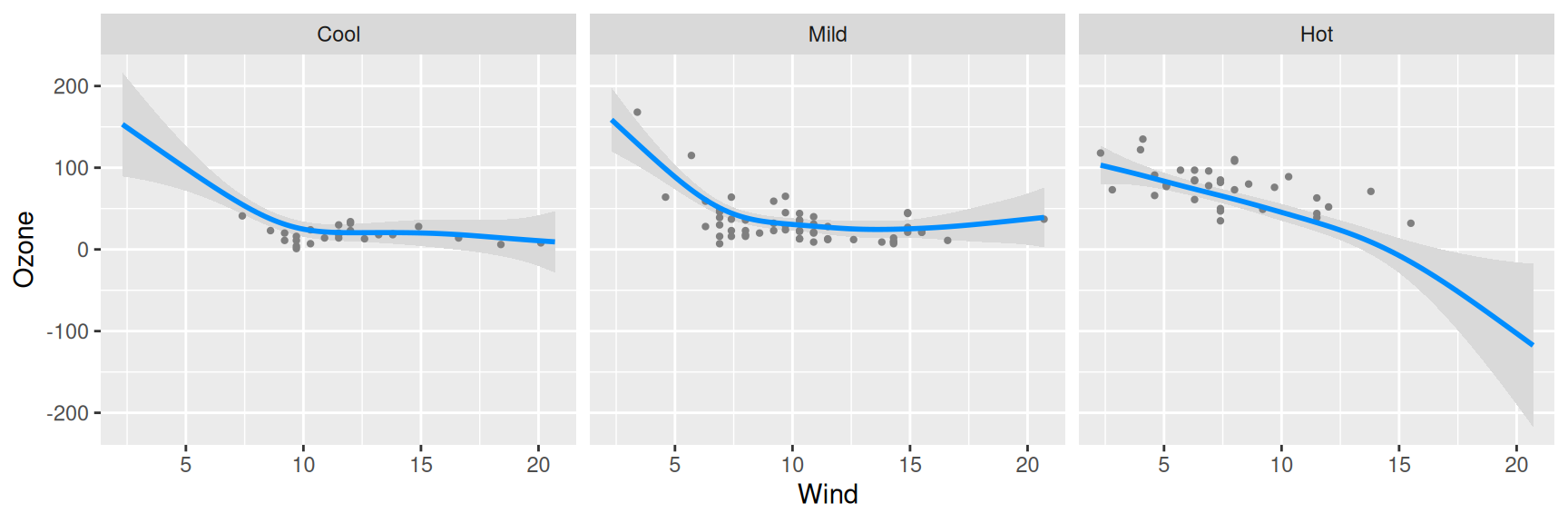visreg is an R package for displaying the results of a fitted model in terms of how a predictor variable x affects an outcome y. The implementation of visreg takes advantage of object-oriented programming in R, meaning that it works with virtually any type of formula-based model in R provided that the model class provides a predict() method: lm, glm, gam, rlm, nlme, lmer, coxph, svm, randomForest and many more.

## Installation

To install the latest release version from CRAN:

install.packages("visreg")

To install the latest development version from GitHub:

remotes::install_github("pbreheny/visreg")

## Usage

The basic usage is that you fit a model, for example:

fit <- lm(Ozone ~ Solar.R + Wind + Temp, data=airquality)

and then you pass it to visreg:

visreg(fit, "Wind")A more complex example, which uses the gam() function from mgcv:

airquality$Heat <- cut(airquality$Temp, 3, labels=c("Cool", "Mild", "Hot"))
fit <- gam(Ozone ~ s(Wind, by=Heat, sp=0.1), data=airquality)
visreg(fit, "Wind", "Heat", gg=TRUE, ylab="Ozone")A number of options for surface plots are also provided, such as rgl plots:

library(splines)
fit <- lm(Ozone ~ Solar.R +ns(Wind, df=2)*ns(Temp, df=2), data=airquality)
visreg2d(fit, "Wind", "Temp", plot.type="rgl")

If your browser supports it, you should be able to interact with above figure (click and drag to rotate).

If you have a question or feature request, please submit an issue.